Data collected 2/10/22

library(pacman)
library(dplyr)
library(tidyverse)
library(jsonlite)
library(xtable)
library(data.table)

### Import Data

# Read the text file from JATOS ...
# ... split it into lines ...
str_split('\n') %>% first() %>%
# ... filter empty rows ...
# ... parse JSON into a data.frame
map_dfr(fromJSON, flatten=T) -> all_data

### Demographics

library(tidyr)

demographics <- all_data %>%
filter(trial_type == "survey-html-form") %>%
select(ID,response) %>%
unnest_wider(response) %>%
mutate(age = as.numeric(age))

age_demographics <- demographics %>%
summarize(mean_age = mean(age),
sd_age = sd(age),
min_age = min(age),
max_age = max(age))

factor_demographics <- apply(demographics[-1], 2, table)

A total of 45 participants were recruited from Amazon’s Mechanical Turk. Mean age was 37.9 (range = 25 to 65 ). There were 11 females, and 34 males. There were 42 right-handed participants, and NA left or both handed participants. 36 participants reported normal vision, and 8 participants reported corrected-to-normal vision. 41 participants reported English as a first language, and 4 participants reported English as a second language.

### Pre-processing

We are interested in including participants who attempted to perform the task to the best of their ability. We adopted the following exclusion criteria.

1. Lower than 75% correct during the encoding task. This means that participants failed to correctly press the F or R keys on each trial.
# select data from the study phase
study_accuracy <- all_data %>%
filter(experiment_phase == "study",
is.na(correct) == FALSE) %>%
group_by(ID)%>%
summarize(mean_correct = mean(correct))

study_excluded_subjects <- study_accuracy %>%
filter(mean_correct < .75) %>%
pull(ID)

ggplot(study_accuracy, aes(x=mean_correct))+
coord_cartesian(xlim=c(0,1))+
geom_vline(xintercept=.75)+
geom_histogram()+
ggtitle("Histogram of mean correct responses \n for each subject during study phase")1. More than 25% Null responses (120*.25 = 30) during test. NULL responses mean that the participant did not respond on a test trial after 10 seconds.
# select data from the study phase
test_null <- all_data %>%
filter(experiment_phase == "test",
response =="NULL") %>%
group_by(ID) %>%
count()

test_null_excluded <- test_null %>%
filter(n > (120*.25)) %>%
pull(ID)

ggplot(test_null, aes(x=n))+
geom_vline(xintercept=30)+
geom_histogram()+
ggtitle("Histogram of count of null responses \n for each subject during test")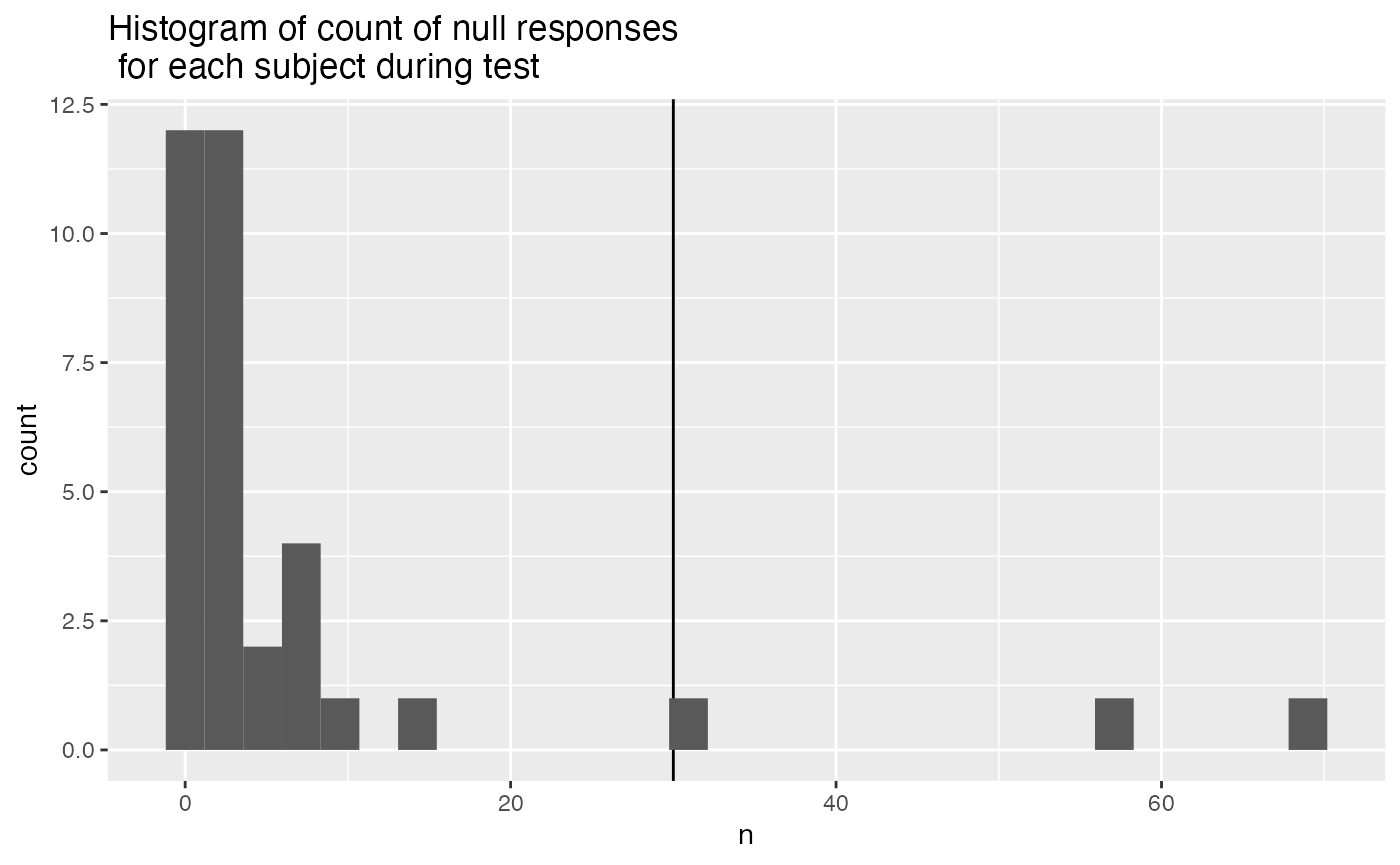1. Higher than 75% response bias in the recognition task. This suggests that participants were simply pressing the same button on most trials.
test_response_bias <- all_data %>%
filter(experiment_phase == "test",
response !="NULL") %>%
mutate(response = as.numeric(response)) %>%
group_by(ID, response) %>%
count() %>%
pivot_wider(names_from = response,
values_from = n,
values_fill = 0) %>%
mutate(bias = abs(0 - 1)/120)

test_response_bias_excluded <- test_response_bias %>%
filter(bias > .75) %>%
pull(ID)

ggplot(test_response_bias, aes(x=bias))+
geom_vline(xintercept=.75)+
geom_histogram()+
ggtitle("Histogram of response bias \n for each subject during test phase")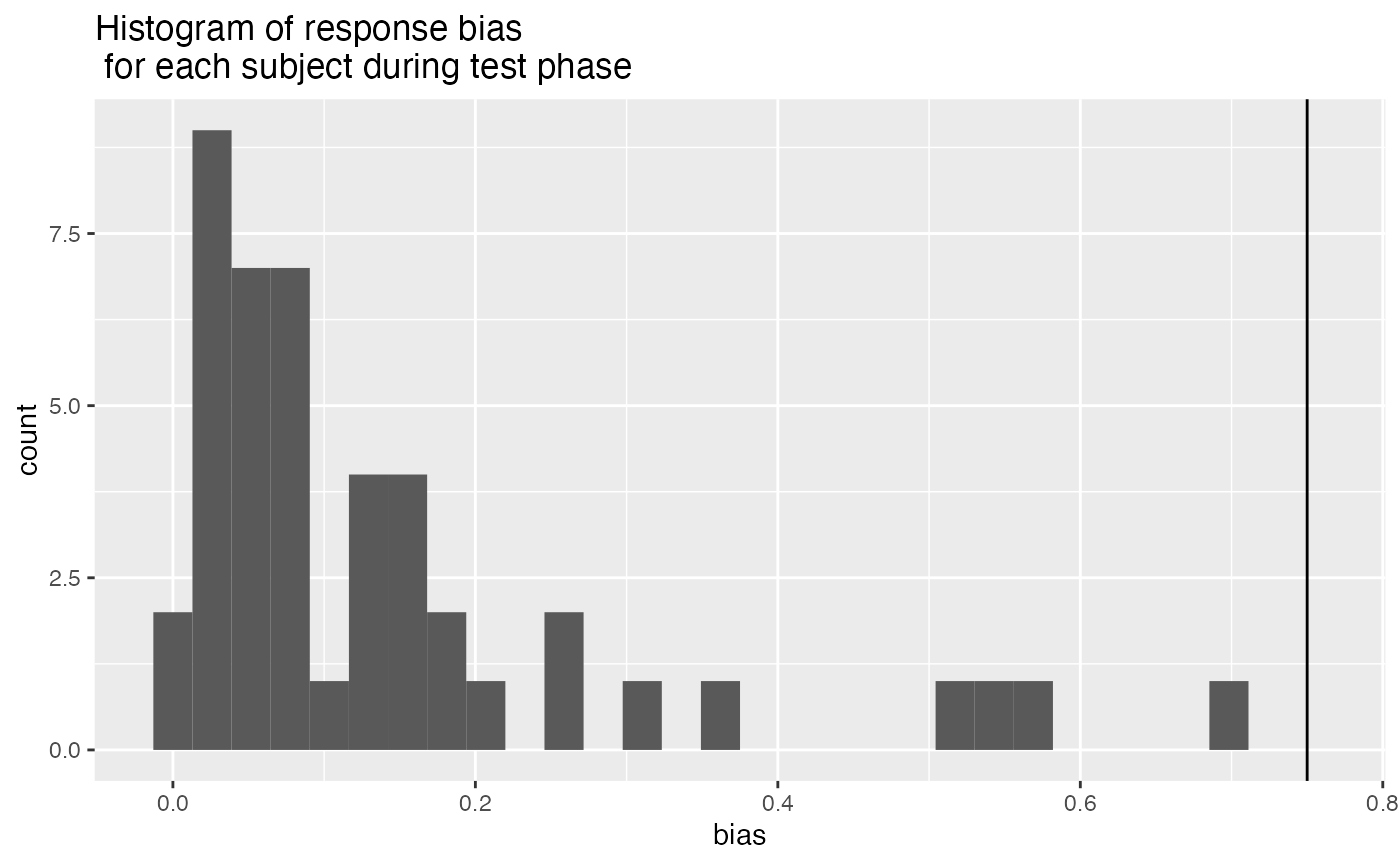1. Making responses too fast during the recognition memory test, indicating that they weren’t performing the task. We excluded participants whose mean RT was less than 300 ms.
test_mean_rt <- all_data %>%
filter(experiment_phase == "test",
response !="NULL",
rt != "NULL") %>%
mutate(rt = as.numeric(rt)) %>%
group_by(ID) %>%
summarize(mean_RT = mean(rt))

test_mean_rt_excluded <- test_mean_rt %>%
filter(mean_RT < 300) %>%
pull(ID)

ggplot(test_mean_rt, aes(x=mean_RT))+
geom_vline(xintercept=300)+
geom_histogram()+
ggtitle("Histogram of response bias \n for each subject during test phase")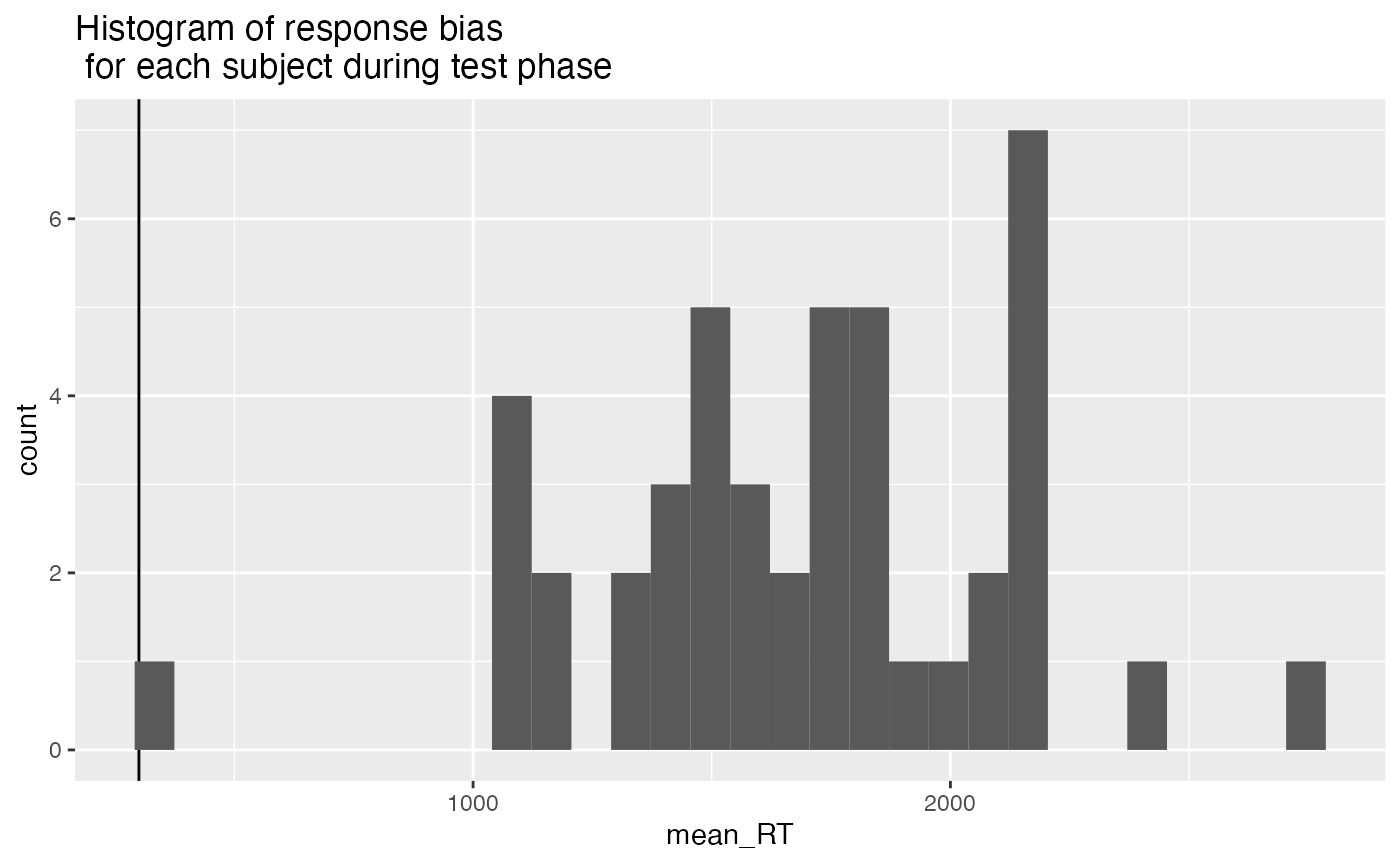1. Subjects are included if they perform better than 55% correct on the novel lures.
test_mean_novel_accuracy <- all_data %>%
filter(experiment_phase == "test",
test_condition == "novel") %>%
mutate(correct = as.logical(correct)) %>%
group_by(ID) %>%
summarize(mean_correct = mean(correct))

test_mean_novel_accuracy_excluded <- test_mean_novel_accuracy %>%
filter(mean_correct < .4) %>%
pull(ID)

ggplot(test_mean_novel_accuracy, aes(x=mean_correct))+
geom_vline(xintercept=.4)+
geom_histogram()+
ggtitle("Histogram of mean accuracy for novel lures \n for each subject during test phase")### All exclusions

all_excluded <- unique(c(study_excluded_subjects,
test_null_excluded,
test_response_bias_excluded,
test_mean_rt_excluded,
test_mean_novel_accuracy_excluded))

length(all_excluded)
##  6

Our participants were recruited online and completed the experiment from a web browser. Our experiment script requests that participants attempt the task to the best of their ability. Nevertheless, it is possible that participants complete the experiment and submit data without attempting to complete the task as directed. We developed a set of criteria to exclude participants whose performance indicated they were not attempting the task as instructed. These criteria also allowed us to confirm that the participants we included in the analysis did attempt the task as instructed to the best of their ability. We adopted the following five criteria:

First, during the encoding phase participants responded to each instructional cue (to remember or forget the picture on each trial) by pressing “R” or “F” on the keyboard. This task demand further served as an attentional check. We excluded participants who scored lower than 75% correct on instructional cue identification responses. Second, participants who did not respond on more than 25% of trials in the recognition test were excluded. Third, we measured response bias (choosing the left or right picture) during the recognition test, and excluded participants who made 75% of their responses to one side (indicating they were repeatedly pressing the same button on each trial). Fourth, we excluded participants whose mean reaction time during the recognition test was less than 300ms, indicating they were pressing the buttons as fast as possible without making a recognition decision. Finally, we computed mean accuracy for the novel lure condition for all participants, and excluded participants whose mean accuracy was less than 55% for those items. All together 6 participants were excluded.

## Accuracy analysis

### Define Helper functions

To do, consider moving the functions into the R package for this project

# attempt general solution

## Declare helper functions

################
# get_mean_sem
# data = a data frame
# grouping_vars = a character vector of factors for analysis contained in data
# dv = a string indicated the dependent variable colunmn name in data
# returns data frame with grouping variables, and mean_{dv}, sem_{dv}
# note: dv in mean_{dv} and sem_{dv} is renamed to the string in dv

get_mean_sem <- function(data, grouping_vars, dv, digits=3){
a <- data %>%
group_by_at(grouping_vars) %>%
summarize("mean_{ dv }" := round(mean(.data[[dv]]), digits),
"sem_{ dv }" := round(sd(.data[[dv]])/sqrt(length(.data[[dv]])),digits),
.groups="drop")
return(a)
}

################
# get_effect_names
# grouping_vars = a character vector of factors for analysis
# returns a named list
# list contains all main effects and interaction terms
# useful for iterating the computation means across design effects and interactions

get_effect_names <- function(grouping_vars){
effect_names <- grouping_vars
if( length(grouping_vars > 1) ){
for( i in 2:length(grouping_vars) ){
effect_names <- c(effect_names,apply(combn(grouping_vars,i),2,paste0,collapse=":"))
}
}
effects <- strsplit(effect_names, split=":")
names(effects) <- effect_names
return(effects)
}

################
# print_list_of_tables
# table_list = a list of named tables
# each table is printed
# names are header level 3

print_list_of_tables <- function(table_list){
for(i in 1:length(table_list)){
cat("###",names(table_list[i]))
cat("\n")
print(knitr::kable(table_list[[i]]))
cat("\n")
}
}

### Conduct Analysis

# create list to hold results
Accuracy <- list()

# Pre-process data for analysis
# assign to "filtered_data" object
Accuracy$filtered_data <- all_data %>% filter(experiment_phase == "test", ID %in% all_excluded == FALSE) # declare factors, IVS, subject variable, and DV Accuracy$factors$IVs <- c("encoding_stimulus_time", "encoding_instruction", "test_condition") Accuracy$factors$subject <- "ID" Accuracy$factors$DV <- "correct" ## Subject-level means used for ANOVA # get individual subject means for each condition Accuracy$subject_means <- get_mean_sem(data=Accuracy$filtered_data, grouping_vars = c(Accuracy$factors$subject, Accuracy$factors$IVs), dv = Accuracy$factors$DV) ## Condition-level means # get all possible main effects and interactions Accuracy$effects <- get_effect_names(Accuracy$factors$IVs)

Accuracy$means <- lapply(Accuracy$effects, FUN = function(x) {
get_mean_sem(data=Accuracy$filtered_data, grouping_vars = x, dv = Accuracy$factors$DV) }) ## ANOVA # ensure factors are factor class Accuracy$subject_means <- Accuracy$subject_means %>% mutate_at(Accuracy$factors$IVs,factor) %>% mutate_at(Accuracy$factors$subject,factor) # run ANOVA Accuracy$aov.out <- aov(mean_correct ~ encoding_stimulus_time*encoding_instruction*test_condition + Error(ID/(encoding_stimulus_time*encoding_instruction*test_condition)), Accuracy$subject_means) # save printable summaries Accuracy$apa_print <- papaja::apa_print(Accuracy$aov.out) ### Graphs Accuracy$graphs$figure <- ggplot(Accuracy$means$encoding_stimulus_time:encoding_instruction:test_condition, aes(x=test_condition, y=mean_correct, group=encoding_instruction, fill=encoding_instruction))+ geom_bar(stat="identity", position="dodge")+ geom_errorbar(aes(ymin = mean_correct-sem_correct, ymax = mean_correct+sem_correct), width=.9, position=position_dodge2(width = 0.2, padding = 0.8))+ facet_wrap(~encoding_stimulus_time)+ coord_cartesian(ylim=c(.4,1))+ geom_hline(yintercept=.5)+ scale_y_continuous(breaks = seq(0.4,1,.1))+ theme_classic(base_size=12)+ ylab("Proportion Correct")+ xlab("Lure Type")+ scale_fill_discrete(name = " Encoding \n Instruction") + ggtitle("E2: Proportion Correct by Stimulus Encoding Duration, \n Encoding Instruction, and Lure Type") Accuracy$graphs$figure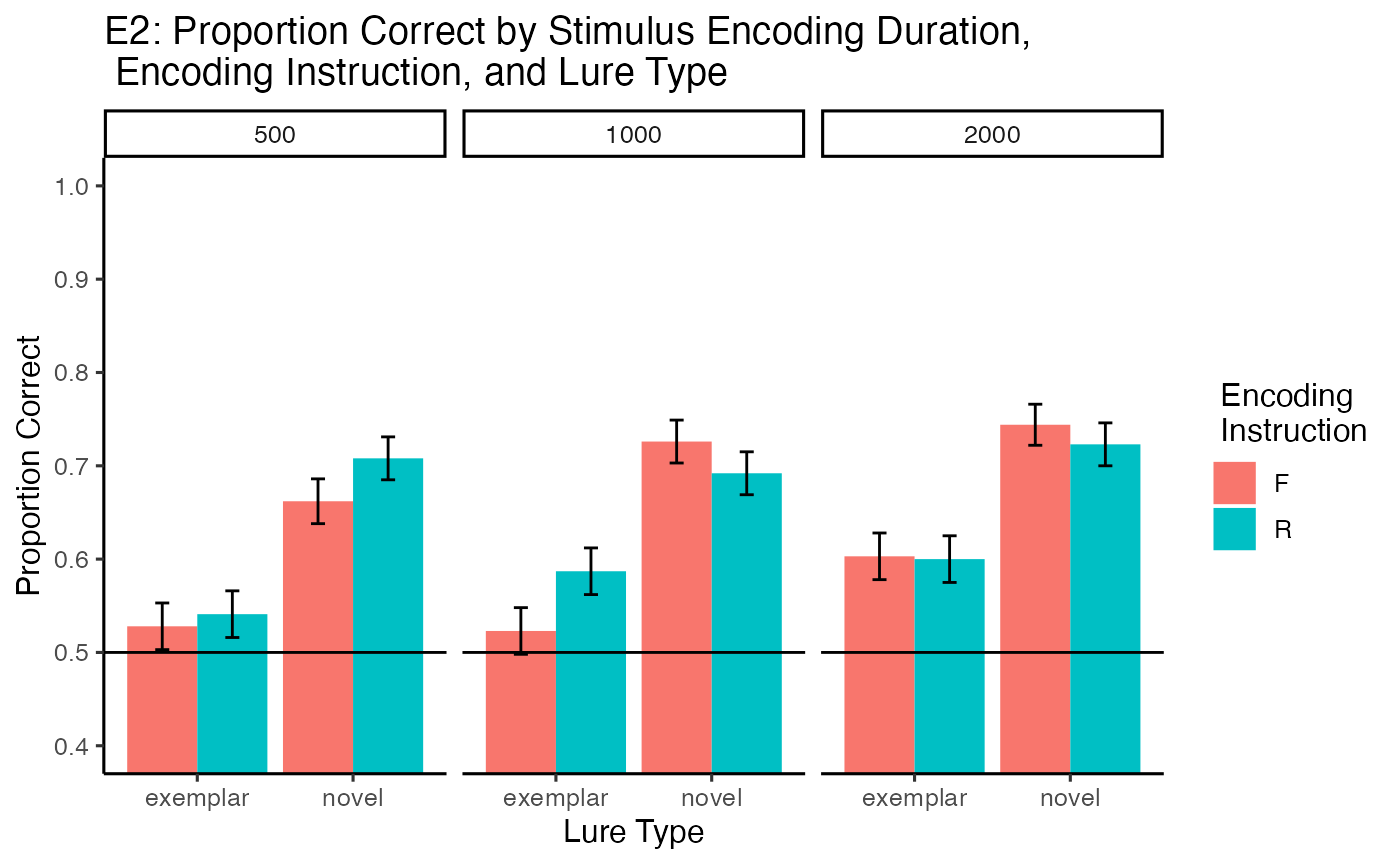knitr::kable(xtable(summary(Accuracy$aov.out)))
Df Sum Sq Mean Sq F value Pr(>F)
Residuals 38 6.4974786 0.1709863 NA NA
encoding_stimulus_time 2 0.2638889 0.1319444 5.5368098 0.0056915
Residuals 76 1.8111111 0.0238304 NA NA
encoding_instruction 1 0.0144444 0.0144444 0.6504279 0.4249786
Residuals 38 0.8438889 0.0222076 NA NA
test_condition 1 2.4700855 2.4700855 79.6634145 0.0000000
Residuals 38 1.1782479 0.0310065 NA NA
encoding_stimulus_time:encoding_instruction 2 0.0338889 0.0169444 0.8266762 0.4413962
Residuals 76 1.5577778 0.0204971 NA NA
encoding_stimulus_time:test_condition 2 0.0105556 0.0052778 0.2553041 0.7753426
Residuals 76 1.5711111 0.0206725 NA NA
encoding_instruction:test_condition 1 0.0218803 0.0218803 1.1289967 0.2946956
Residuals 38 0.7364530 0.0193803 NA NA
encoding_stimulus_time:encoding_instruction:test_condition 2 0.0846581 0.0423291 2.4242561 0.0953629
Residuals 76 1.3270085 0.0174606 NA NA
print_list_of_tables(Accuracy$means) #### encoding_stimulus_time encoding_stimulus_time mean_correct sem_correct 500 0.610 0.012 1000 0.632 0.012 2000 0.667 0.012 #### encoding_instruction encoding_instruction mean_correct sem_correct F 0.631 0.01 R 0.642 0.01 #### test_condition test_condition mean_correct sem_correct exemplar 0.564 0.010 novel 0.709 0.009 #### encoding_stimulus_time:encoding_instruction encoding_stimulus_time encoding_instruction mean_correct sem_correct 500 F 0.595 0.018 500 R 0.624 0.017 1000 F 0.624 0.017 1000 R 0.640 0.017 2000 F 0.673 0.017 2000 R 0.662 0.017 #### encoding_stimulus_time:test_condition encoding_stimulus_time test_condition mean_correct sem_correct 500 exemplar 0.535 0.018 500 novel 0.685 0.017 1000 exemplar 0.555 0.018 1000 novel 0.709 0.016 2000 exemplar 0.601 0.018 2000 novel 0.733 0.016 #### encoding_instruction:test_condition encoding_instruction test_condition mean_correct sem_correct F exemplar 0.551 0.015 F novel 0.710 0.013 R exemplar 0.576 0.014 R novel 0.708 0.013 #### encoding_stimulus_time:encoding_instruction:test_condition encoding_stimulus_time encoding_instruction test_condition mean_correct sem_correct 500 F exemplar 0.528 0.025 500 F novel 0.662 0.024 500 R exemplar 0.541 0.025 500 R novel 0.708 0.023 1000 F exemplar 0.523 0.025 1000 F novel 0.726 0.023 1000 R exemplar 0.587 0.025 1000 R novel 0.692 0.023 2000 F exemplar 0.603 0.025 2000 F novel 0.744 0.022 2000 R exemplar 0.600 0.025 2000 R novel 0.723 0.023 ### Comparisons ## Encoding time x instruction Accuracy$simple$DF_500 <- Accuracy$subject_means %>%
filter(encoding_stimulus_time == "500") %>%
group_by(ID,encoding_instruction) %>%
summarize(mean_correct = mean(mean_correct)) %>%
pivot_wider(names_from = encoding_instruction,
values_from = mean_correct) %>%
mutate(difference = R-F) %>%
pull(difference) %>%
t.test() %>%
papaja::apa_print()

Accuracy$simple$DF_1000 <- Accuracy$subject_means %>% filter(encoding_stimulus_time == "1000") %>% group_by(ID,encoding_instruction) %>% summarize(mean_correct = mean(mean_correct)) %>% pivot_wider(names_from = encoding_instruction, values_from = mean_correct) %>% mutate(difference = R-F) %>% pull(difference) %>% t.test() %>% papaja::apa_print() Accuracy$simple$DF_2000 <- Accuracy$subject_means %>%
filter(encoding_stimulus_time == "2000") %>%
group_by(ID,encoding_instruction) %>%
summarize(mean_correct = mean(mean_correct)) %>%
pivot_wider(names_from = encoding_instruction,
values_from = mean_correct) %>%
mutate(difference = R-F) %>%
pull(difference) %>%
t.test() %>%
papaja::apa_print()

# encoding time x test condition

Accuracy$simple$test_500 <- Accuracy$subject_means %>% filter(encoding_stimulus_time == "500") %>% group_by(ID,test_condition) %>% summarize(mean_correct = mean(mean_correct)) %>% pivot_wider(names_from = test_condition, values_from = mean_correct) %>% mutate(difference = novel-exemplar) %>% pull(difference) %>% t.test() %>% papaja::apa_print() Accuracy$simple$test_1000 <- Accuracy$subject_means %>%
filter(encoding_stimulus_time == "1000") %>%
group_by(ID,test_condition) %>%
summarize(mean_correct = mean(mean_correct)) %>%
pivot_wider(names_from = test_condition,
values_from = mean_correct) %>%
mutate(difference = novel-exemplar) %>%
pull(difference) %>%
t.test() %>%
papaja::apa_print()

Accuracy$simple$test_2000 <- Accuracy$subject_means %>% filter(encoding_stimulus_time == "2000") %>% group_by(ID,test_condition) %>% summarize(mean_correct = mean(mean_correct)) %>% pivot_wider(names_from = test_condition, values_from = mean_correct) %>% mutate(difference = novel-exemplar) %>% pull(difference) %>% t.test() %>% papaja::apa_print() ### Write-up ## helper print functions qprint <- function(data,iv,level,dv){ data[[iv]] %>% filter(.data[[iv]] == {level}) %>% pull(dv) } qprint_mean_sem <- function(data,iv,level,dv){ dv_mean <- data[[iv]] %>% filter(.data[[iv]] == {level}) %>% pull(dv) dv_sem <- data[[iv]] %>% filter(.data[[iv]] == {level}) %>% pull(dv) return(paste("M = ", dv_mean, ", SEM = ", dv_sem, sep="")) } # qprint(Accuracy$means,"encoding_stimulus_time","500","mean_correct")
# qprint_mean_sem(Accuracy$means,"encoding_stimulus_time","500",c("mean_correct","sem_correct")) # use data.table for interactions #t <- as.data.table(Accuracy$means$encoding_stimulus_time:encoding_instruction) #t[encoding_stimulus_time==500 & encoding_instruction == "F"]$mean_correct

Proportion correct for each subject in each condition was submitted to a 3 (Encoding Duration: 500ms, 1000ms, 2000ms) x 2 (Encoding Instruction: Forget vs. Remember) x 2 (Lure type: Novel vs. Exemplar) fully repeated measures ANOVA. For completeness, each main effect and higher-order interaction is described in turn.

The main effect of encoding duration was significant, $$F(2, 76) = 5.54$$, $$p = .006$$, $$\hat{\eta}^2_G = .017$$, 90% CI $$[.000, .074]$$. Proportion correct was lowest for the 500 ms duration (M = 0.61, SEM = 0.012), and higher for the 1000 ms (M = 0.632, SEM = 0.012), and 2000 ms (M = 0.667, SEM = 0.012) stimulus durations.

The main effect of encoding instruction was not significant, $$F(1, 38) = 0.65$$, $$p = .425$$, $$\hat{\eta}^2_G = .001$$, 90% CI $$[.000, .055]$$. Proportion correct was similar for remember cues (M = 0.642, SEM = 0.01) and forget cues (M = 0.631, SEM = 0.01).

The main effect of lure type was significant, $$F(1, 38) = 79.66$$, $$p < .001$$, $$\hat{\eta}^2_G = .137$$, 90% CI $$[.014, .312]$$. Proportion correct was higher for novel lures (M = 0.709, SEM = 0.009) than exemplar lures (M = 0.564, SEM = 0.01).

The main question of interest was whether directing forgetting would vary across the encoding duration times. The interaction between encoding instruction and encoding duration was not significant, $$F(2, 76) = 0.83$$, $$p = .441$$, $$\hat{\eta}^2_G = .002$$, 90% CI $$[.000, .013]$$.

Paired sample t-tests were used to assess the directed forgetting effect at each encoding duration. The directed forgetting effect is taken as the difference between proportion correct for remember minus forget items. At 500 ms, the directed forgetting effect was not significant, $$M = 0.03$$, 95% CI $$[-0.01, 0.07]$$, $$t(38) = 1.36$$, $$p = .181$$. At 1000ms, the directed forgetting effect was not significant, $$M = 0.02$$, 95% CI $$[-0.03, 0.06]$$, $$t(38) = 0.63$$, $$p = .531$$. And, at 2000 ms, the directed forgetting effect was again not detected, $$M = -0.01$$, 95% CI $$[-0.06, 0.04]$$, $$t(38) = -0.49$$, $$p = .629$$.

The encoding duration by lure type interaction was not significnat, $$F(2, 76) = 0.26$$, $$p = .775$$, $$\hat{\eta}^2_G = .001$$, 90% CI $$[.000, .000]$$. The encoding instruction by lure type interaction was not significant, $$F(1, 38) = 1.13$$, $$p = .295$$, $$\hat{\eta}^2_G = .001$$, 90% CI $$[.000, .065]$$. Similarly, the interaction between encoding duration, instruction, and lure type was not significant, $$F(2, 76) = 2.42$$, $$p = .095$$, $$\hat{\eta}^2_G = .005$$, 90% CI $$[.000, .037]$$.

## Reaction Time Analysis

### Conduct Analysis

# create list to hold results
RT <- list()

# Pre-process data for analysis
# assign to "filtered_data" object
RT$filtered_data <- all_data %>% filter(experiment_phase == "test", ID %in% all_excluded == FALSE, rt != "NULL") %>% mutate(rt = as.numeric(rt)) # declare factors, IVS, subject variable, and DV RT$factors$IVs <- c("encoding_stimulus_time", "encoding_instruction", "test_condition") RT$factors$subject <- "ID" RT$factors$DV <- "rt" ## Subject-level means used for ANOVA # get individual subject means for each condition RT$subject_means <- get_mean_sem(data=RT$filtered_data, grouping_vars = c(RT$factors$subject, RT$factors$IVs), dv = RT$factors$DV) ## Condition-level means # get all possible main effects and interactions RT$effects <- get_effect_names(RT$factors$IVs)

RT$means <- lapply(RT$effects, FUN = function(x) {
get_mean_sem(data=RT$filtered_data, grouping_vars = x, dv = RT$factors$DV) }) ## ANOVA # ensure factors are factor class RT$subject_means <- RT$subject_means %>% mutate_at(RT$factors$IVs,factor) %>% mutate_at(RT$factors$subject,factor) # run ANOVA RT$aov.out <- aov(mean_rt ~ encoding_stimulus_time*encoding_instruction*test_condition + Error(ID/(encoding_stimulus_time*encoding_instruction*test_condition)), RT$subject_means) # save printable summaries RT$apa_print <- papaja::apa_print(RT$aov.out) ### Graphs RT$graphs$figure <- ggplot(RT$means$encoding_stimulus_time:encoding_instruction:test_condition, aes(x=test_condition, y=mean_rt, group=encoding_instruction, fill=encoding_instruction))+ geom_bar(stat="identity", position="dodge")+ geom_errorbar(aes(ymin = mean_rt-sem_rt, ymax = mean_rt+sem_rt), width=.9, position=position_dodge2(width = 0.2, padding = 0.8))+ facet_wrap(~encoding_stimulus_time)+ coord_cartesian(ylim=c(1000,2000))+ scale_y_continuous(breaks = seq(1000,2000,100))+ theme_classic(base_size=12)+ ylab("Mean RT (ms)")+ xlab("Lure Type")+ scale_fill_discrete(name = " Encoding \n Instruction") + ggtitle("E2: Mean RT by Stimulus Encoding Duration, \n Encoding Instruction, and Lure Type") RT$graphs$figure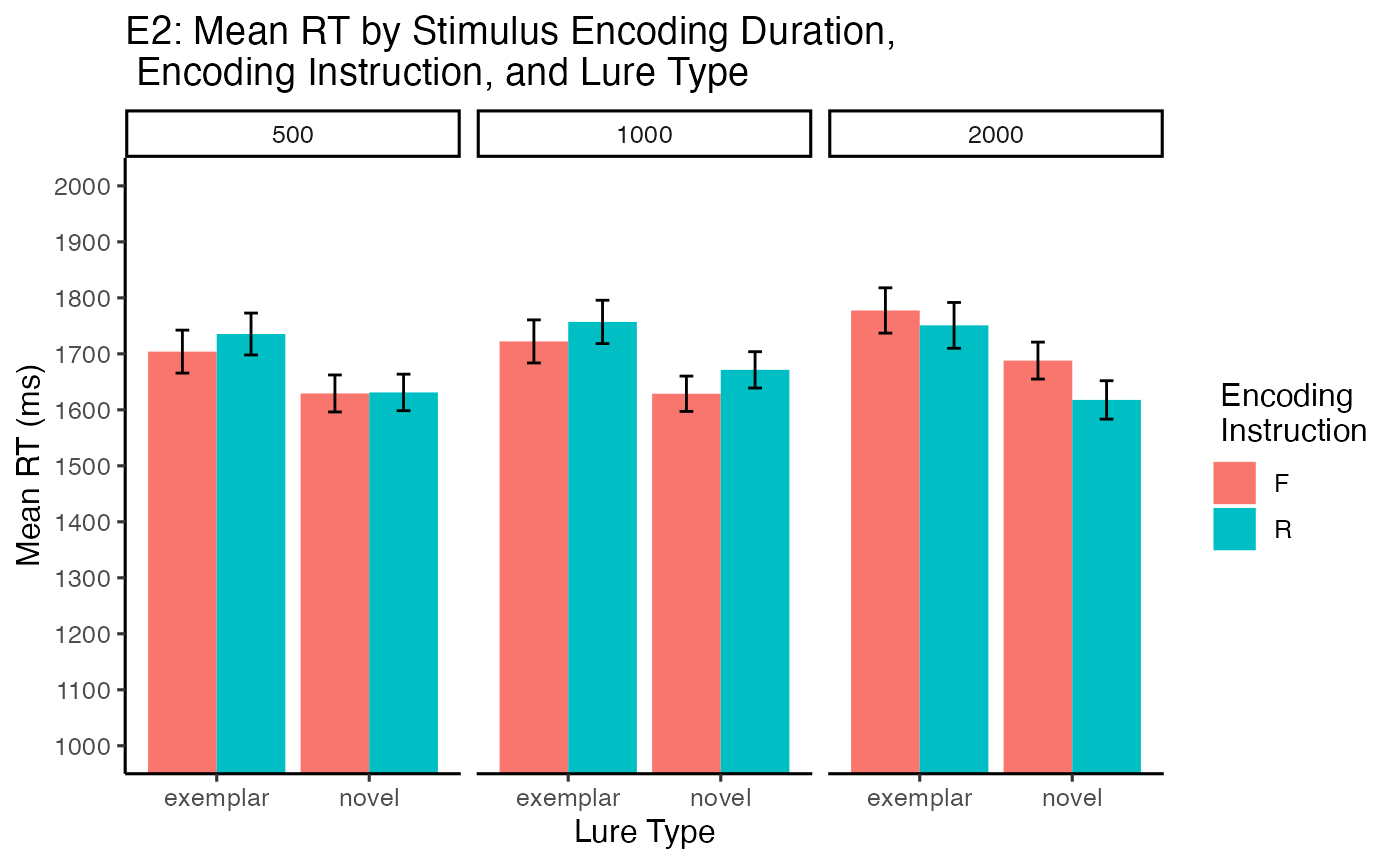knitr::kable(xtable(summary(RT$aov.out)))
Df Sum Sq Mean Sq F value Pr(>F)
Residuals 38 5.837090e+07 1536076.4179 NA NA
encoding_stimulus_time 2 7.792940e+04 38964.7013 1.0603772 0.3513923
Residuals 76 2.792702e+06 36746.0764 NA NA
encoding_instruction 1 4.961696e+02 496.1696 0.0109629 0.9171616
Residuals 38 1.719847e+06 45259.1256 NA NA
test_condition 1 1.060501e+06 1060500.6813 7.3165610 0.0101686
Residuals 38 5.507919e+06 144945.2390 NA NA
encoding_stimulus_time:encoding_instruction 2 1.463802e+05 73190.0810 2.7237547 0.0720377
Residuals 76 2.042198e+06 26871.0254 NA NA
encoding_stimulus_time:test_condition 2 1.788846e+04 8944.2308 0.2383893 0.7884828
Residuals 76 2.851476e+06 37519.4245 NA NA
encoding_instruction:test_condition 1 1.626283e+04 16262.8313 0.4717691 0.4963449
Residuals 38 1.309937e+06 34472.0134 NA NA
encoding_stimulus_time:encoding_instruction:test_condition 2 2.239374e+04 11196.8723 0.3467641 0.7080848
Residuals 76 2.454009e+06 32289.5985 NA NA
print_list_of_tables(RT$means) #### encoding_stimulus_time encoding_stimulus_time mean_rt sem_rt 500 1674.869 17.745 1000 1694.952 17.753 2000 1708.100 18.705 #### encoding_instruction encoding_instruction mean_rt sem_rt F 1691.484 14.709 R 1693.774 14.805 #### test_condition test_condition mean_rt sem_rt exemplar 1741.122 15.939 novel 1644.224 13.401 #### encoding_stimulus_time:encoding_instruction encoding_stimulus_time encoding_instruction mean_rt sem_rt 500 F 1666.543 25.333 500 R 1683.227 24.867 1000 F 1675.428 24.909 1000 R 1714.350 25.297 2000 F 1732.740 26.145 2000 R 1683.651 26.740 #### encoding_stimulus_time:test_condition encoding_stimulus_time test_condition mean_rt sem_rt 500 exemplar 1719.656 26.787 500 novel 1630.139 23.192 1000 exemplar 1739.679 27.266 1000 novel 1650.050 22.626 2000 exemplar 1764.156 28.758 2000 novel 1652.480 23.817 #### encoding_instruction:test_condition encoding_instruction test_condition mean_rt sem_rt F exemplar 1734.456 22.578 F novel 1648.512 18.783 R exemplar 1747.777 22.513 R novel 1639.959 19.124 #### encoding_stimulus_time:encoding_instruction:test_condition encoding_stimulus_time encoding_instruction test_condition mean_rt sem_rt 500 F exemplar 1703.950 38.374 500 F novel 1629.232 33.038 500 R exemplar 1735.404 37.414 500 R novel 1631.051 32.599 1000 F exemplar 1722.168 38.455 1000 F novel 1628.687 31.542 1000 R exemplar 1757.009 38.692 1000 R novel 1671.357 32.451 2000 F exemplar 1777.441 40.468 2000 F novel 1687.923 32.988 2000 R exemplar 1750.837 40.914 2000 R novel 1617.678 34.287 ### Comparisons ## Encoding time x instruction RT$simple$DF_500 <- RT$subject_means %>%
filter(encoding_stimulus_time == "500") %>%
group_by(ID,encoding_instruction) %>%
summarize(mean_rt = mean(mean_rt)) %>%
pivot_wider(names_from = encoding_instruction,
values_from = mean_rt) %>%
mutate(difference = R-F) %>%
pull(difference) %>%
t.test() %>%
papaja::apa_print()

RT$simple$DF_1000 <- RT$subject_means %>% filter(encoding_stimulus_time == "1000") %>% group_by(ID,encoding_instruction) %>% summarize(mean_rt = mean(mean_rt)) %>% pivot_wider(names_from = encoding_instruction, values_from = mean_rt) %>% mutate(difference = R-F) %>% pull(difference) %>% t.test() %>% papaja::apa_print() RT$simple$DF_2000 <- RT$subject_means %>%
filter(encoding_stimulus_time == "2000") %>%
group_by(ID,encoding_instruction) %>%
summarize(mean_rt = mean(mean_rt)) %>%
pivot_wider(names_from = encoding_instruction,
values_from = mean_rt) %>%
mutate(difference = R-F) %>%
pull(difference) %>%
t.test() %>%
papaja::apa_print()

# encoding time x test condition

RT$simple$test_500 <- RT$subject_means %>% filter(encoding_stimulus_time == "500") %>% group_by(ID,test_condition) %>% summarize(mean_rt = mean(mean_rt)) %>% pivot_wider(names_from = test_condition, values_from = mean_rt) %>% mutate(difference = novel-exemplar) %>% pull(difference) %>% t.test() %>% papaja::apa_print() RT$simple$test_1000 <- RT$subject_means %>%
filter(encoding_stimulus_time == "1000") %>%
group_by(ID,test_condition) %>%
summarize(mean_rt = mean(mean_rt)) %>%
pivot_wider(names_from = test_condition,
values_from = mean_rt) %>%
mutate(difference = novel-exemplar) %>%
pull(difference) %>%
t.test() %>%
papaja::apa_print()

RT$simple$test_2000 <- RT\$subject_means %>%
filter(encoding_stimulus_time == "2000") %>%
group_by(ID,test_condition) %>%
summarize(mean_rt = mean(mean_rt)) %>%
pivot_wider(names_from = test_condition,
values_from = mean_rt) %>%
mutate(difference = novel-exemplar) %>%
pull(difference) %>%
t.test() %>%
papaja::apa_print()

### Write-up

Mean reaction times on correct trials for each subject in each condition were submitted to a 3 (Encoding Duration: 500ms, 1000ms, 2000ms) x 2 (Encoding Instruction: Forget vs. Remember) x 2 (Lure type: Novel vs. Exemplar) fully repeated measures ANOVA. For brevity we report only the significant effects. The full analysis is contained in supplementary materials.

The main effect of lure type was significant, $$F(1, 38) = 7.32$$, $$p = .010$$, $$\hat{\eta}^2_G = .014$$, 90% CI $$[.000, .128]$$. Mean reaction times were faster in the novel lure condition (M = 1644.224, SEM = 13.401) than exemplar lure condition (M = 1741.122, SEM = 15.939).

The remaining main effects and interactions were not significant.

### save environment

save.image("data/E2/E2_data_write_up.RData")